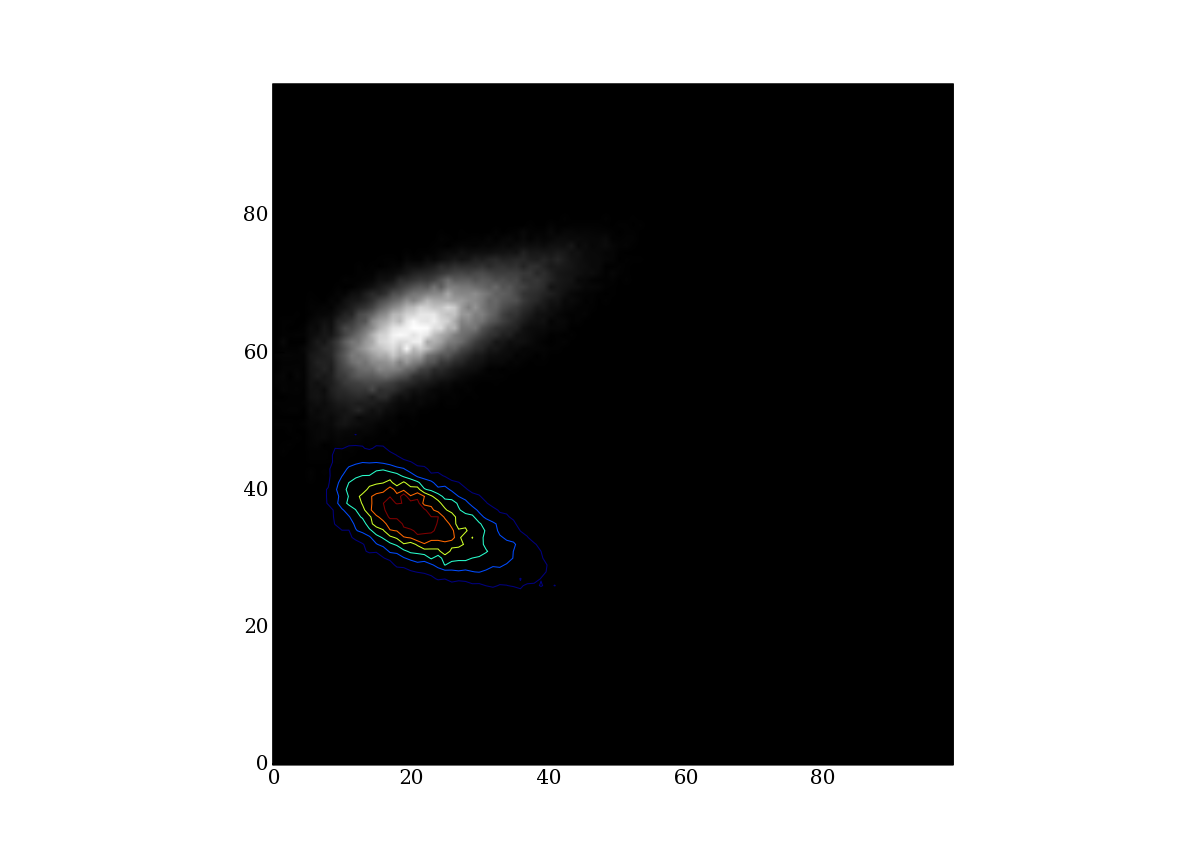# contour bug?

I thing there are a small problem with contour.

import pyfits
import pylab
data = pyfits.getdata('test.fits')
pylab.imshow(data,cmap=cm.gray)
pylab.contour(data)
#to have the result wanted:
pylab.contour(data,origin='upper')
pylab.show()

not sure that it's something did in purpose.

Thanks,

N.test.fits (81.6 KB)

humufr@...136... wrote:

I thing there are a small problem with contour.

import pyfits
import pylab
data = pyfits.getdata('test.fits')
pylab.imshow(data,cmap=cm.gray)
pylab.contour(data)
#to have the result wanted:
pylab.contour(data,origin='upper')
pylab.show()

not sure that it's something did in purpose.

It is intentional, not a bug. See examples/contour_image.py

Eric

So that means that contour does have origin set a lower by default when imshow
is set at upper? If it's intentional I'll note it but I'm must admit that I
have a problem with the logic.

N.

···

Le lundi 16 octobre 2006 15:55, Eric Firing a écrit :

humufr@...136... wrote:
> I thing there are a small problem with contour.
>
> import pyfits
> import pylab
> data = pyfits.getdata('test.fits')
> pylab.imshow(data,cmap=cm.gray)
> pylab.contour(data)
> #to have the result wanted:
> pylab.contour(data,origin='upper')
> pylab.show()
>
> not sure that it's something did in purpose.

It is intentional, not a bug. See examples/contour_image.py

Eric

humufr@...136... wrote:

I thing there are a small problem with contour.

import pyfits
import pylab
data = pyfits.getdata('test.fits')
pylab.imshow(data,cmap=cm.gray)
pylab.contour(data)
#to have the result wanted:
pylab.contour(data,origin='upper')
pylab.show()

not sure that it's something did in purpose.

It is intentional, not a bug. See examples/contour_image.py

Eric

So that means that contour does have origin set a lower by default when imshow is set at upper? If it's intentional I'll note it but I'm must admit that I have a problem with the logic.

It is more a matter of convention than logic. To the extent that there is logic, it might run like this:

An image is a string of bytes representing pixels arranged like letters in text, starting from the upper left.

When no explicit X and Y vectors are given, contour and pcolor are implicitly using the array indices as X and Y.

Eric

···

Le lundi 16 octobre 2006 15:55, Eric Firing a écrit :

humufr@...136... wrote: